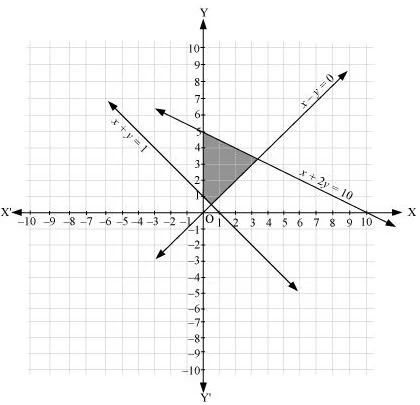# Class 11 Maths NCERT Solutions for Chapter 6 Linear Inequalities Exercise 6.3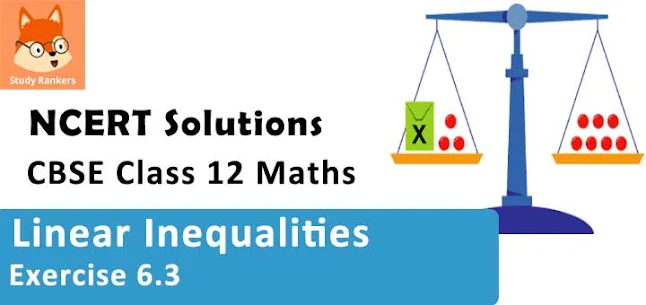### Linear Inequalities Exercise 6.3 Solutions

1. Solve the following system of inequalities graphically: x ≥ 3, y ≥ 2.

Solution

x ≥ 3 …(1)
y ≥ 2 …(2)
The graph of the lines, x = 3 and y = 2, are drawn in the figure below.
Inequality (1) represents the region on the right hand side of the line, x = 3 (including the line x = 3), and inequality (2) represents the region above the line, = 2 (including the line = 2).
Hence, the solution of the given system of linear inequalities is represented by the common shaded region including the points on the respective lines as follows.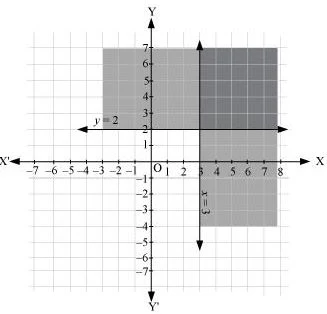2. Solve the following system of inequalities graphically: 3x + 2y ≤ 12, x ≥ 1, y ≥ 2

Solution

3x + 2y ≤ 12 …(1)
x ≥ 1 …(2)
y ≥ 2 …(3)
The graphs of the lines, 3x + 2y = 12, x = 1, and y = 2, are drawn in the figure below.
Inequality (1) represents the region below the line, 3x + 2y = 12 (including the line 3x + 2y = 12). Inequality (2) represents the region on the right side of the line, x = 1 (including the line x = 1). Inequality (3) represents the region above the line, y = 2 (including the line y = 2).
Hence, the solution of the given system of linear inequalities is represented by the common shaded region including the points on the respective lines as follows.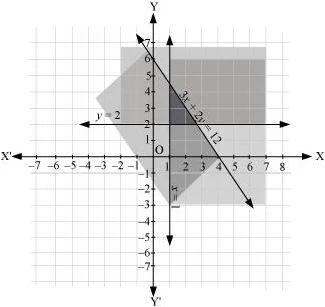3. Solve the following system of inequalities graphically: 2x + y≥ 6, 3x + 4y ≤ 12

Solution

2x + y≥ 6 …(1)
3x + 4y ≤ 12 …(2)
The graph of the lines, 2x + y= 6 and 3x + 4= 12, are drawn in the figure below.
Inequality (1) represents the region above the line, 2x + y= 6 (including the line 2x + y= 6), and inequality (2) represents the region below the line, 3x + 4y =12 (including the line 3x + 4y =12).
Hence, the solution of the given system of linear inequalities is represented by the common shaded region including the points on the respective lines as follows.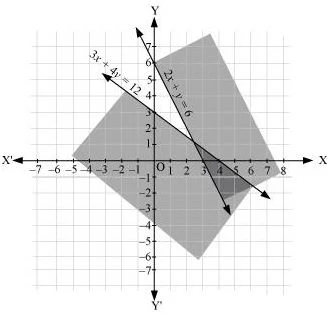4. Solve the following system of inequalities graphically: x + y≥ 4, 2x – y > 0

Solution

x + y≥ 4 …(1)
2x – y > 0 …(2)
The graph of the lines, x + y = 4 and 2x – y = 0, are drawn in the figure below.
Inequality (1) represents the region above the line, x + y = 4 (including the line x + y = 4).
It is observed that (1, 0) satisfies the inequality, 2x – y > 0. [2(1) – 0 = 2 > 0]
Therefore, inequality (2) represents the half plane corresponding to the line, 2x – y = 0, containing the point (1, 0) [excluding the line 2x – y > 0].
Hence, the solution of the given system of linear inequalities is represented by the common shaded region including the points on line x + y = 4 and excluding the points on line 2x – y = 0 as follows.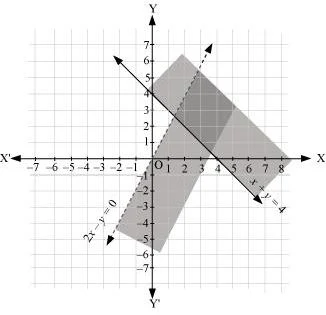5. Solve the following system of inequalities graphically: 2x – y > 1, x – 2y < –1

Solution

2x – y > 1 …(1)
x – 2y < –1 …(2)
The graph of the lines, 2x – y = 1 and x – 2= –1, are drawn in the figure below.
Inequality (1) represents the region below the line, 2x – y = 1 (excluding the line 2x – y = 1), and inequality (2) represents the region above the line, x – 2= –1 (excluding the line x – 2= –1).
Hence, the solution of the given system of linear inequalities is represented by the common shaded region excluding the points on the respective lines as follows.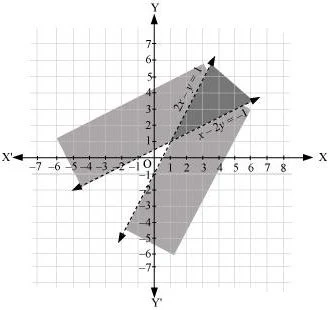6. Solve the following system of inequalities graphically: x + y ≤ 6, x + y ≥ 4

Solution

x + y ≤ 6 …(1)
x + y ≥ 4 …(2)
The graph of the lines, x + y = 6 and x + y = 4, are drawn in the figure below.
Inequality (1) represents the region below the line, x + y = 6 (including the line x + y = 6), and inequality (2) represents the region above the line, x + y = 4 (including the line x + y = 4).
Hence, the solution of the given system of linear inequalities is represented by the common shaded region including the points on the respective lines as follows.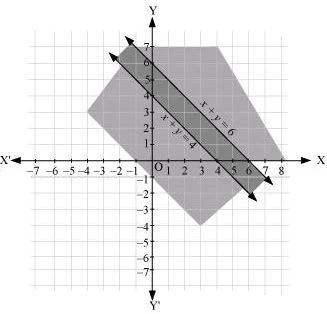7. Solve the following system of inequalities graphically: 2x + y≥ 8, x + 2y ≥ 10

Solution

2x + y= 8 …(1)
x + 2= 10 …(2)
The graph of the lines, 2x + y= 8 and x + 2= 10, are drawn in the figure below.
Inequality (1) represents the region above the line, 2x + = 8, and inequality (2) represents the region above the line, x + 2= 10.
Hence, the solution of the given system of linear inequalities is represented by the common shaded region including the points on the respective lines as follows.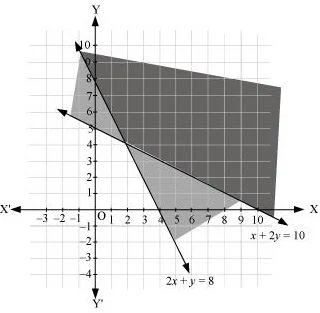8. Solve the following system of inequalities graphically: x + y ≤ 9, y > xx ≥ 0

Solution

x + y ≤ 9 ...(1)
y > x ...(2)
x ≥ 0 ...(3)
The graph of the lines, x + y= 9 and y = x, are drawn in the figure below.
Inequality (1) represents the region below the line, x + y = 9 (including the line x + y = 9).
It is observed that (0, 1) satisfies the inequality, y > x. [1 > 0]
Therefore, inequality (2) represents the half plane corresponding to the line, y = x, containing the point (0, 1) [excluding the line y = x].
Inequality (3) represents the region on the right hand side of the line, x = 0 or y-axis (including y-axis).
Hence, the solution of the given system of linear inequalities is represented by the common shaded region including the points on the lines, x + y = 9 and x = 0, and excluding the points on line y = x as follows.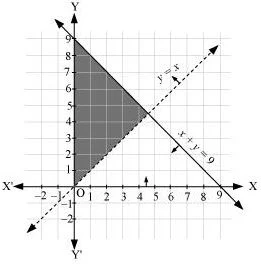9. Solve the following system of inequalities graphically: 5x + 4y ≤ 20, x ≥ 1, y ≥ 2

Solution

5x + 4y ≤ 20 ...(1)
x ≥ 1 …(2)
y ≥ 2 …(3)
The graph of the lines, 5x + 4y = 20, x = 1, and y = 2, are drawn in the figure below.
Inequality (1) represents the region below the line, 5x + 4y = 20 (including the line 5x + 4y = 20). Inequality (2) represents the region on the right hand side of the line, x = 1 (including the line x = 1). Inequality (3) represents the region above the line, y = 2 (including the line y = 2).
Hence, the solution of the given system of linear inequalities is represented by the common shaded region including the points on the respective lines as follows.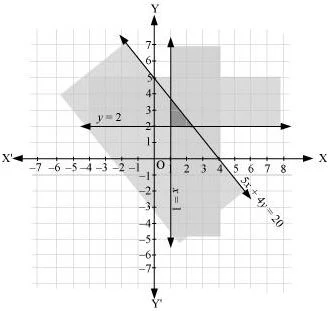10. Solve the following system of inequalities graphically: 3x + 4y ≤ 60, x + 3y ≤ 30, x ≥ 0, y ≥ 0
Solution

3x + 4y ≤ 60 …(1)
x + 3y ≤ 30 …(2)
The graph of the lines, 3x + 4y = 60 and x + 3y = 30, are drawn in the figure below.
Inequality (1) represents the region below the line, 3x + 4y = 60 (including the line 3x + 4y = 60), and inequality (2) represents the region below the line, x + 3y = 30 (including the line x + 3y = 30).
Since x ≥ 0 and y ≥ 0, every point in the common shaded region in the first quadrant including the points on the respective line and the axes represents the solution of the given system of linear inequalities.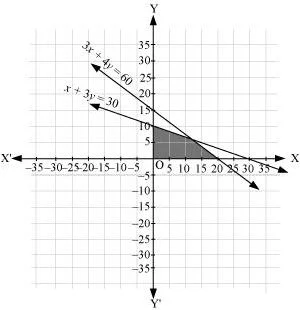11. Solve the following system of inequalities graphically: 2x + y≥ 4, x + y ≤ 3, 2x – 3y ≤ 6

Solution

2x + y≥ 4 …(1)
x + y ≤ 3 …(2)
2x – 3y ≤ 6 …(3)
The graph of the lines, 2x + y= 4, x + y = 3, and 2x – 3y = 6, are drawn in the figure below.
Inequality (1) represents the region above the line, 2x + y= 4 (including the line 2x + y= 4). Inequality (2) represents the region below the line,
x + y = 3 (including the line x + y = 3). Inequality (3) represents the region above the line, 2x – 3y = 6 (including the line 2x – 3y = 6).
Hence, the solution of the given system of linear inequalities is represented by the common shaded region including the points on the respective lines as follows.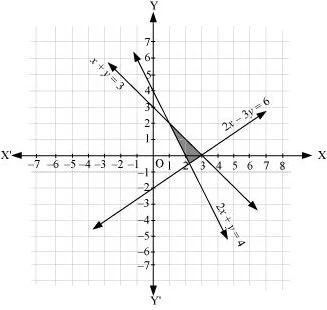12. Solve the following system of inequalities graphically :
x – 2y ≤ 3, 3x + 4y ≥ 12, x ≥ 0, y ≥ 1

Solution

x – 2y ≤ 3 …(1)
3x + 4y ≥ 12 …(2)
y ≥ 1 …(3)
The graph of the lines, x – 2y = 3, 3x + 4y = 12, and y = 1, are drawn in the figure below.
Inequality (1) represents the region above the line, x – 2y = 3 (including the line x – 2y = 3). Inequality (2) represents the region above the line, 3x + 4y = 12 (including the line 3x + 4y = 12). Inequality (3) represents the region above the line, y = 1 (including the line y = 1).
The inequality, x ≥ 0, represents the region on the right hand side of y-axis (including y-axis).
Hence, the solution of the given system of linear inequalities is represented by the common shaded region including the points on the respective lines and y- axis as follows.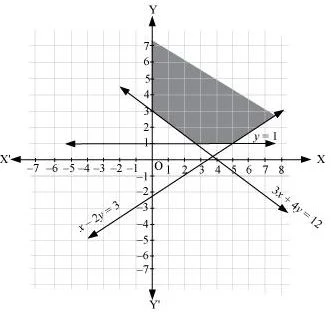13. Solve the following system of inequalities graphically:
4x + 3y ≤ 60, y ≥ 2xx ≥ 3, xy ≥ 0
Solution

4x + 3y ≤ 60 …(1)
y ≥ 2x …(2)
x ≥ 3 …(3)
The graph of the lines, 4x + 3y = 60, y = 2x, and x = 3, are drawn in the figure below.
Inequality (1) represents the region below the line, 4x + 3y = 60 (including the line 4x + 3y = 60). Inequality (2) represents the region above the line, y = 2(including the line y = 2x). Inequality (3) represents the region on the right hand side of the line, x = 3 (including the line x = 3).
Hence, the solution of the given system of linear inequalities is represented by the common shaded region including the points on the respective lines as follows.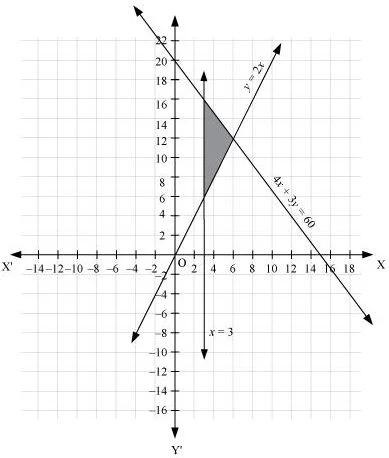14. Solve the following system of inequalities graphically: 3x + 2y ≤ 150, x + 4y ≤ 80, x ≤ 15, y ≥ 0, x ≥ 0

Solution

3x + 2y ≤ 150 …(1)
x + 4y ≤ 80 …(2)
x ≤ 15 …(3)
The graph of the lines, 3x + 2y = 150, x + 4y = 80, and x = 15, are drawn in the figure below.
Inequality (1) represents the region below the line, 3x + 2y = 150 (including the line 3x + 2y = 150). Inequality (2) represents the region below the line, x + 4y = 80 (including the line x + 4y = 80). Inequality (3) represents the region on the left hand side of the line, x = 15 (including the line x = 15).
Since x ≥ 0 and y ≥ 0, every point in the common shaded region in the first quadrant including the points on the respective lines and the axes represents the solution of the given system of linear inequalities.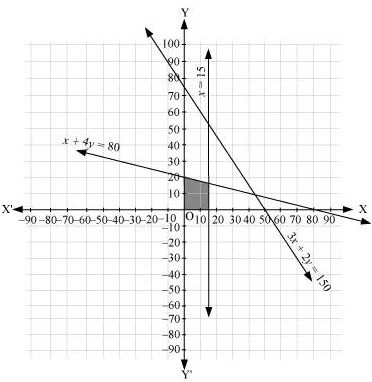15. Solve the following system of inequalities graphically: x + 2y ≤ 10, x + y ≥ 1, x – y ≤ 0, x ≥ 0, y ≥ 0
Solution

x + 2y ≤ 10 …(1)
x + y ≥ 1 …(2)
x – y ≤ 0 …(3)
The graph of the lines, x + 2y = 10, x + y = 1, and x – y = 0, are drawn in the figure below.
Inequality (1) represents the region below the line, x + 2y = 10 (including the line x + 2y = 10). Inequality (2) represents the region above the line, x + y = 1 (including the line x + y = 1). Inequality (3) represents the region above the line, x – y = 0 (including the line x – y = 0).
Since x ≥ 0 and y ≥ 0, every point in the common shaded region in the first quadrant including the points on the respective lines and the axes represents the solution of the given system of linear inequalities.